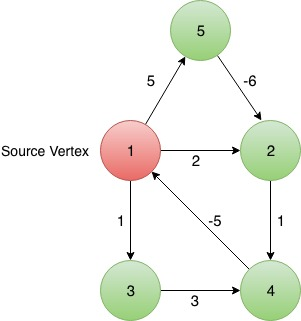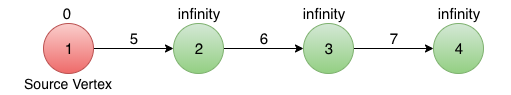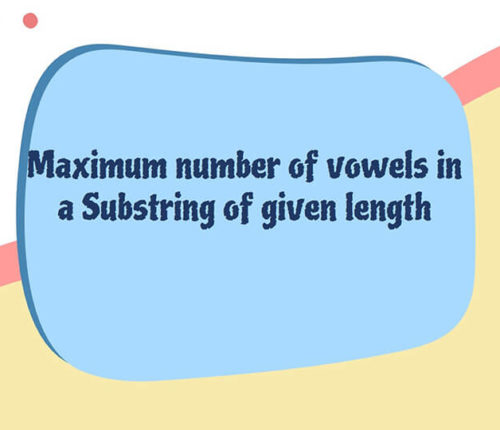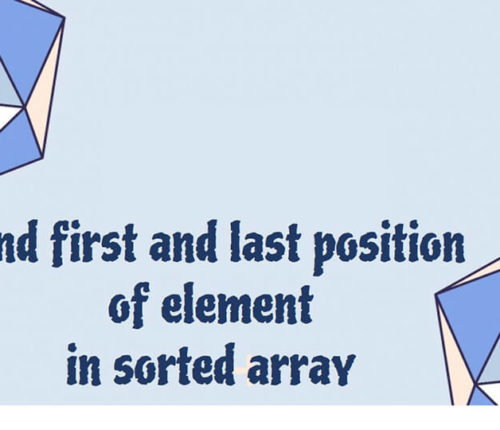# Bellman Ford Algorithm in java

If you want to practice data structure and algorithm programs, you can go through Java coding interview questions.

In this post, we will see about Bellman ford algorithm in java.

`Bellman Ford Algorithm` is used to find shortest Distance of all Vertices from a given source vertex in a Directed Graph. Dijkstra Algorithm also serves the same purpose more efficiently but the Bellman-Ford Algorithm also works for Graphs with Negative weight edges. In addition to that, it also detects if there is any negative Cycle in the graphs.Input Format :
In the first line, given two space separated integers, that is, number of vertices(V) and edges(E).
Next E lines contains three integers each, which represents an edges from first integer to second integer with weight equal to third integer.
In the last line given an integer representing the source.

Output Format:
Output the shortest distance of every vertex from given source.

INPUT-1:
5 7
1 2 2
1 5 5
1 3 1
2 4 1
3 3 4
4 1 5
5 2 -6
1OUTPUT-1:
Distance of 1 from source is 0 and path followed is 1
Distance of 2 from source is -1 and path followed is 1->5->2
Distance of 3 from source is 1 and path followed is 1->3
Distance of 4 from source is 0 and path followed is 1->5->2->4
Distance of 5 from source is 5 and path followed is 1->5INPUT-2:
5 7
1 2 2
1 5 5
1 3 1
2 4 1
3 3 4
4 1 -5
5 2 -6
2OUTPUT-2:
Negative Cycle Detected

Algorithm:
Basically in this algorithm, we are looping in a random set of edges, vertices-1 times.
That random set can be any order of all the edges of the given graph.

• For every edge, E(u->v), we check if the current distance of v from source is greater than the distance of u and the weight of this edge combined, if it is, this means that we just found a better path with lesser distance than the previous path, and we update the distance of Vertex v from source to distance of u + weight of E(u->v).
• Say the random order of edges we will be processing is,
{(1,2), (1,3), (1,5), (2,4), (4,1), (3,4), (5,2)}
We first need to initialize the distance of all the vertices from source vertex to be Infinity except the source vertex itself, as it is 0 distance away from itself.
We need to maintain a HashMap for storing the nodes which contain, name of vertex, distance from source node, path of the shortest distance from source to this vertex. Vertex, Node will be the key value pair for the table.
Now initially our HashMap will be this,
vertex -> (vertex, distance, path)
1 -> (1, 0, 1)
2 -> (2, Infinity, ” “)
3 -> (3, Infinity, ” “)
4 -> (4, Infinity, ” “)
5 -> (5, Infinity, ” “)
• Now we process this set of edges (V-1) times, that is, 4 times,

First iteration :

Here distance(x) represents shortest distance of vertex x from source node till that iteration.

edge(1->2), distance(2) > distance(1) + weight(1,2), distance(2) will be updated to 0 + 2 = 2 and the path of vertex 2 will now be, path of vertex 1 + "2", that is, 1->2

edge(1->3), distance(3) > distance(1) + weight(1,3), distance(3) will be updated to 0 + 1 = 1 and the path of vertex 3 will now be, path of vertex 1 + "3", that is, 1->3

edge(1->5), distance(5) > distance(1) + weight(1,5), distance(5) will be updated to 0 + 5 = 5
and the path of vertex 5 will now be, path of vertex 1 + "5", that is, 1->5

edge(2->4), distance(4) > distance(2) + weight(2,4), distance(4) will be updated to 2 + 1 = 3
and the path of vertex 4 will now be, path of vertex 2 + "4", that is, 1->2->4

edge(4->1), distance(1) < distance(4) + weight(4,1), distance(1) will not be updated and hence no updation in their path as well. edge(3->4), distance(4) < distance(3) + weight(3,4), distance(4) will not be updated and hence no updation in their path as well. edge(5->2), distance(2) > distance(5) + weight(5,2), distance(2) will be updated to 5+(-6)= -1 and the path of vertex 2 will now be, path of vertex 1 + "2", that is, 1->5->2.

Second iteration :
edge(1->2), distance(2) = distance(1) + weight(1,2), distance(2) will not be updated and hence no updation in their path as well.

edge(1->3), distance(3) = distance(1) + weight(1,3), distance(3) will not be updated and hence no updation in their path as well.

edge(2->4), distance(4) > distance(2) + weight(2,4), distance(4) will be updated to -1 + 1 = 0
and the path of vertex 4 will now be, path of vertex 2 + "4", that is, 1->5->2->4

edge(3->4), distance(4) < distance(3) + weight(3,4), distance(4) will not be updated and hence no updation in their path as well. edge(5->2), distance(2) = distance(5) + weight(5,2), distance(2) will not be updated and hence no updation in their path as well.

Third iteration :

No edge(u->v) will hold the condition distance(v)>distance(u)+weight(v, u).

Fourth and (vertices-1)th iteration :

No updation.

• After (Vertices – 1) iterations are done, we iterate the same list one more time to check if there is a negative cycle or not.
During this very last iteration, if there is updation in the shortest distance again, this means that there is definitely a negative cycle in the graph, and hence, the shortest distance of every vertex can not be calculated for this graph as we can keep looping again and again in that negative cycle to keep reducing our distance further. Thus, we print that the graph contains a negative cycle and return from the function.

We can clearly have the doubt of why do we need to iterate (vertices – 1) times, when we can achieve the shortest distance in less than (V-1) iterations.

The number of iterations it will take to find out the shortest distance for every vertex, completely depends on the random order in which we are processing our edges. Remember, I am emphasising on finding out shortest distance for calculating which in the worst case we need to iterate atleast          (vertices – 1) times.

Lets see,
What if we had the order of the set starting with farthest(in terms of edges) from the source node, we would try to check if dist(2) > dist(5) + weight(2,5), but the correct distance of 5 isn’t calculated yet and it is still Infinity, so we do nothing, and now we move to second edge whose correct distance again isn’t calculated yet, so we do nothing again, so ultimately after this iteration end we will be able to update distance of only those vertices which are immediate neighbour of source.

To understand this more clearly, lets consider the worst possible random set of edges for this linear graph,• Say the random order of edges we will be processing is,
{(1,2), (2,3), (3,4)}
Now in the first Iteration,
=> for (1,2), distance(1) > distance(2) + weight(1,2), therefore, distance(2) gets updated to 5 from          Infinity.
=> for (2,3), distance(3) > distance(2) + weight(2,3), distance(3) is now 5+6 = 11.
=> for (3,4), distance(4) > distance(3) + weight(2,3), distance(3) is now 11+7 = 18.

We see that the shortest possible distance of all the vertices(2,3,4) from source(1) got calculated in just one iteration.

• Now lets consider a different Random order of edges,
{(3,4), (2,3), (1,2)}

In the first iteration, no updation will be seen for any edge except the immediate neighbour of source vertex, because while calculating the distance for vertex 4, distance of vertex 2 hasn’t been calculated yet. Same will be the case while calculating for vertex 3. Distance of a farther vertex can’t be calculated until we know the distance(doesn’t matter if it’s smallest or not) of closer vertex.

In second iteration, no updation will be there for vertex 4, as the distance of closer vertex 3 hasn’t been calculated yet. So updation will be seen for vertex 3 as we already calculated a distance of vertex 2 from source vertex.

And now in the third and (vertices-1)th iteration, we finally find the distance for vertex 4 because now we know the distance of vertex 3 from source vertex.

• There we see, number of iterations totally depends on the random order in which we process the edges, but in the worst random set of edges, we needed to iterate (V-1) times to completely calculate the shortest distance of all vertices from given source node. And hence we have our answer for the question, Why do we do this process for a random set of edges for Vertices-1 times?

Number of iterations = (Vertices – 1) + 1{one more iteration for detecting negative cycle}
= Vertices
and every time we are processing a set containing all of the Edges.
Therefore, the worst time Complexity for this algorithm will be O(V*E).

That’s all about Bellman ford algorithm in java.

import_contacts

## Related Posts

•18 June

### Maximum Number of Vowels in a Substring of Given Length

Table of ContentsApproach – 1 Generate All Substrings Using substring() MethodApproach – 2 Using Sliding Window Method (Linear Time Solution) In this article, we will look at an interesting problem related to the Strings and [Sliding-Window Algorithm](https://java2blog.com/sliding-window-maximum-java/ “Sliding-Window Algorithm”). The problem is : "Given a String we have to Find the Maximum Number of Vowel […]

•04 June

### Search for a range Leetcode – Find first and last position of element in sorted array

Table of ContentsApproach 1 (Using Linear Search)Approach 2 (Using Modified Binary Search-Optimal) In this article, we will look into an interesting problem asked in Coding Interviews related to Searching Algorithms. The problem is: Given a Sorted Array, we need to find the first and last position of an element in Sorted array. This problem is […]

•30 April

### Convert Postfix to Infix in Java

Learn about how to convert Postfix to Infix in java.

•30 April

### Convert Prefix to Postfix in Java

Learn about how to convert Prefix to Postfix in java.

•16 April

### Data Structures in java

•29 November

### Top 100+ Java coding interview questions

Table of ContentsStringQuestion 1 : How to reverse a String in java? Can you write a program without using any java inbuilt methods?Question 2 : Write a java program to check if two Strings are anagram in java?Question 3 : Write a program to check if String has all unique characters in java?Question 4 : […]

1.Karthik says: